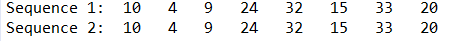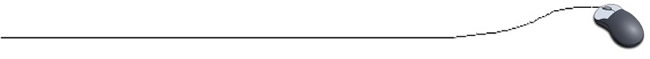Random Number Generation

Computers can be used to simulate the generation of random numbers. This random generation is referred to as a pseudo-random generation.  These created values are not truly "random" because a mathematical formula is used to generate the values.

The "random" numbers generated by the mathematical algorithm are given a starting number (called the "seed") and always generates the same sequence of numbers.  Since the same sequence is generated each time the seed remains the same, the sequence of random numbers is referred to as being a pseudo-random sequence.  To prevent the same sequence from being generated each time, the "seed" value must be changed.

We have already seen the Math.random() method in the Math class which returns a random floating point number (double) between 0 and 1.

 To generate random integer numbers between 1 and 30 inclusive: int number = (int) (Math.random() * 30 + 1);
Behind the scenes: The double number between 0 and 1 (0.0 - 0.999999) gets multiplied by 30, giving numbers between 0.0 and 29.99997. Add 1 to get 1.0 to 30.99997. The casting to int, truncates the values to 1 to 30.# We are now going to take a look at theRandom class.

 java.util.Random class creates random int, double, and float values (plus others).

 Random()     constructs a Random object using the current time as the "seed" Random(seed)      constructs a Random object using a specified seed nextInt()      returns a random int value starting at 0 nextInt(n)     returns a random int value between 0 and integer n (but not n) nextDouble()      returns a double value between 0.0 and 1.0 (but not 1.0)
NOTE: The "current time" used by Random() is the time in milliseconds since January 1, 1970.
Fortunately, this seed will be continually changing, giving you different numbers with each run.

Example: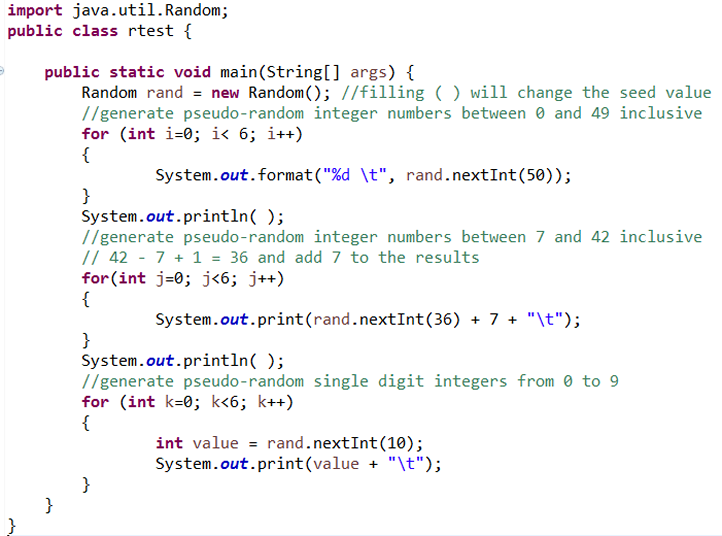Output: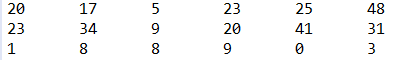If the same "seed" value is specified, the sequence of psuedo-random numbers will be the same. This example shows the generation of 2 sets of 8 random integer numbers from 0 to 34 inclusive, where the random seed is set at 55 for both sets. Notice that the sets are identical. This example shows that the Java generation of random numbers is actually pseudo-random generation since it can be controlled by a formula.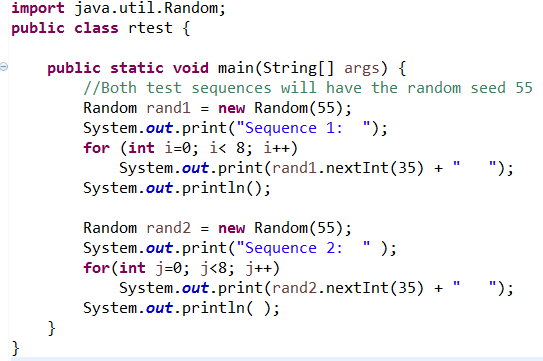Output: